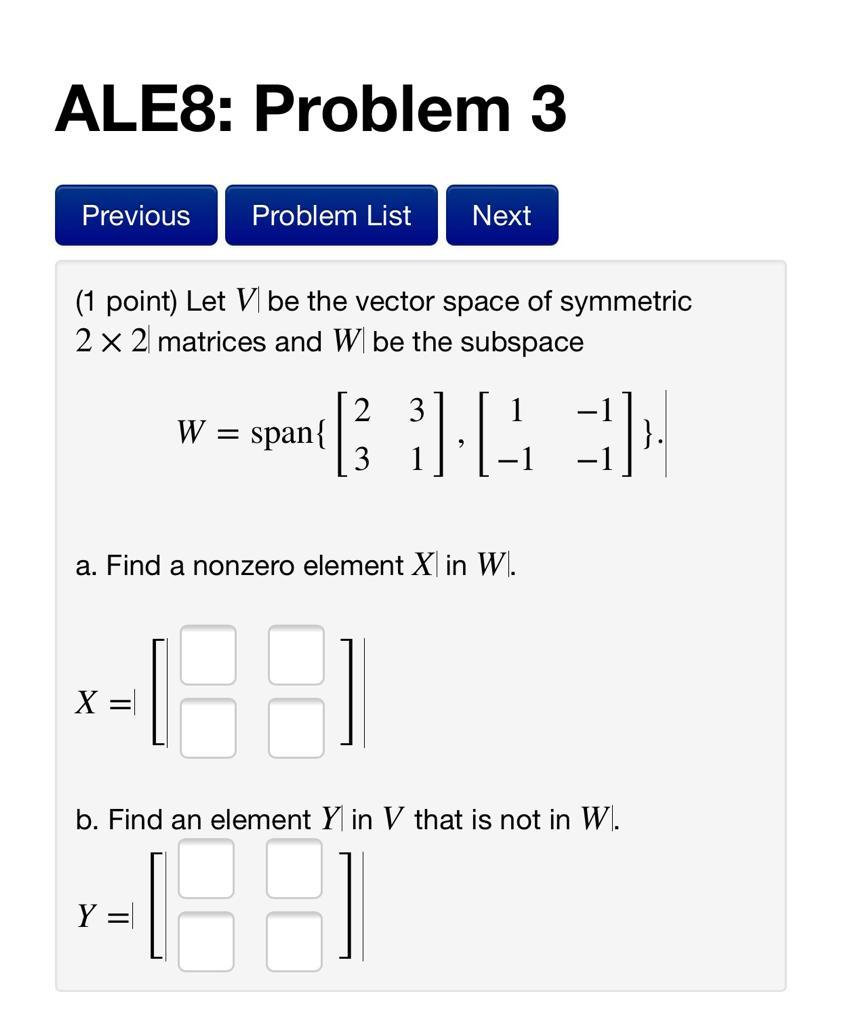### Create an Account

Already have account?

### Forgot Your Password ?

Home / Questions / ALES: Problem 3 Previous Problem List Next (1 point) Let Vl be the vector space of symmetr...

# ALES: Problem 3 Previous Problem List Next (1 point) Let Vl be the vector space of symmetric 2 x 2 matrices and W be the subspace W = span{ }. a. Find a nonzero element X in W. -=[FI CH b. Find an ele

ALES: Problem 3 Previous Problem List Next (1 point) Let Vl be the vector space of symmetric 2 x 2 matrices and W be the subspace W = span{ }. a. Find a nonzero element X in W. -=[FI CH b. Find an element Y in V that is not in W. Y=Apr 15 2021 View more View Less

#### Answer (Solved)Subscribe To Get Solution